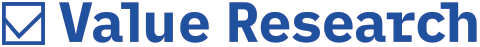# Check the expiry date of your debt fund

##### Average portfolio maturity helps an investor know how sensitive a debt fund is to changes in interest ratesAs we all know, debt funds invest in bonds and securities which mature over a certain period of time. The maturity is the length of the period at the end of which the principal amount is returned to the security-holder or bond-holder. Since a debt fund invests in a number of such instruments, each of these instruments would have a different maturity. So the fund calculates a weighted average maturity, which gives a fair idea of the fund's maturity period. For example, if a fund owns three bonds of two-year (Rs 30,000), three-year (Rs 10,000) and five-year (Rs 20,000) maturities, its weighted average maturity would be 3.17 years.

So why does average maturity matter? It is all very well to calculate the weighted average maturity, but one also needs to understand what it entails. A fund's average maturity is important because it tells you how sensitive a fund is to a change in interest rates. It is also important to keep in mind that changes in interest rates affect different securities differently. The price of long-term debt securities generally fluctuates more than that of short-term securities when the interest rate changes. Consequently, mutual funds with several long-maturity papers in their portfolio are more sensitive to net asset value (NAV) fluctuations.

For example, among all debt funds in the Medium to Long Duration category, UTI Bond Fund has the highest average maturity (10.44 years as on Aug 19, 2020). As a result, this fund's standard deviation - a measure of a fund's volatility - is also on the higher side at 5.89. On the other hand, ICICI Prudential Debt Management Fund has an average maturity of just 0 years, with a standard deviation of 1.43, indicating less volatility. You can find the Average Portfolio Maturity on VRO in two ways. You can either visit an individual fund page or you can use our Fund Selector tool. In both cases, you will find it under the Portfolio tab (as shown below).

The average maturity of a portfolio changes with time and also whenever the portfolio is churned. As a debt security approaches its maturity date, the length of time to maturity becomes shorter. Thus, even if a fund buys and holds a debt portfolio, the average maturity of a fund keeps on decreasing till the security held reaches its maturity date.

Also, if a fund sells one security and buys a new one, its average maturity will change. Gilt funds usually have a relatively high average maturity and are the most volatile among all debt funds. Cash funds like liquid and overnight funds, on the other hand, have the shortest average maturity and are the least volatile. But we usually associate high risk with higher returns and hence, gilt funds are capable of delivering higher returns than other debt funds. So if you are an aggressive investor, you know that gilt funds would suit your needs while cash funds are for the more conservative investor.